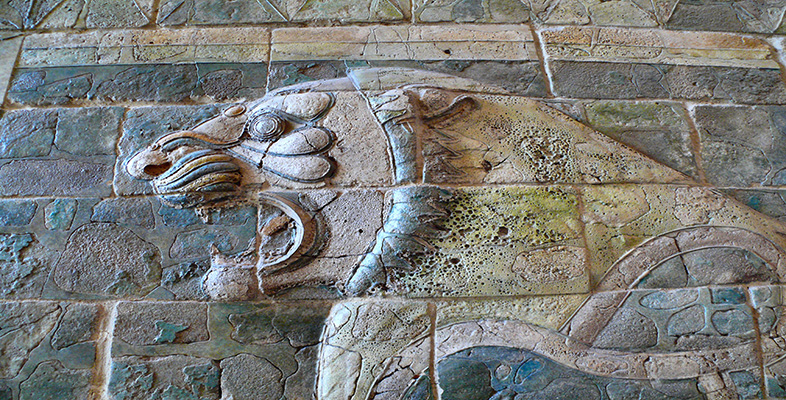Babylonian mathematics

Start this free course now. Just create an account and sign in. Enrol and complete the course for a free statement of participation or digital badge if available.

Free course

# 1.6 The social context of Babylonian mathematical activity

The extant mathematical tablets from the Old Babylonian period fall broadly into two categories, table texts and problem texts. You have seen examples of both of these. The weighing-the-stone problem with which we started is from a problem text, while all the others—the table of squares, the reciprocal table and Plimpton 322—are table texts, tablets consisting solely of tables of numbers. Several hundred table texts have been found, and many types of calculations appear to have been carried out by means of them. As well as squares and reciprocals, there are multiplication tables, tables of cubes and cube roots, tables of the sums of squares and cubes, combined tables where several of these are present, tables for working out compound interest, tables of weights and measures, and others. Numerical tables seem to have been a staple constituent of Babylonian life, as ubiquitous for them as is the pocket calculator for us today. Problem texts, by contrast, are rarer—only a hundred or so tablets have been found—and they seem to relate to an educational context of advanced scribal training. Early Mesopotamian culture had seen the development of specialised occupations, as a part of the newly-developing and highly-complex urban structuring of the community, and the profession of scribe was central to the running of economic, bureaucratic and other aspects of the state. There were special institutions, schools, for training future scribes in the arts of writing, counting and accounting, and other necessary skills. (The attached pdf affords revealing glimpses into the scribal art and its training.)

Scribal art and its training [Tip: hold Ctrl and click a link to open it in a new tab. (Hide tip)]

It is in this educational context that the problem texts seem to have been written and used. Some merely give the problem and the answer (the one you saw in Question 1 was of this sort); others are fortunately more forthcoming on what to do to reach the answer. Let us look at one of these now.

Please read through the extract below to gain an impression of its style.

I have subtracted the side of my square for the area: 14, 30. You write down 1, the coefficient. You break off half of 1. 0; 30 and 0; 30 you multiply. You add 0; 15 to 14, 30. Result 14, 30; 15. This is the square of 29; 30. You add 0; 30, which you multiplied, to 29; 30. Result 30, the side of the square.

The problem is given in the first sentence, the rest is its solution. It turns out that after doing various things to the numbers initially given, the result 30 is reached. This indeed solves the original problem, for a square of side 30 has area 302 = 900 (= 15,0 in sexagesimal), and subtracting 30 from 900 gives 870 (= 14,30). This is a problem of the kind we would call quadratic, that is, involving the square of some unknown number which is to be found. As a way in to understanding the Babylonian computational procedure for this problem, let us follow the instructions through in a modern algebraic format (and take into account later how far this may have distorted the Babylonian scribe's activity).

Let us call the unknown, the side, ‘x’; the coefficient, (which here is 1), call ‘b’; and the number in the statement of the problem (here, 14,30) call ‘c’. Then the problem is; to find x, where x2bx = c. The solution consists of taking the coefficient b, halving it, ½b squaring that, (½b)2; adding this to the result, c+(½b)2; taking the square root of that, √(c + (½b)2); and finally adding the result to the halved coefficient ½b + √(c + (½b)2); which is the answer. Indeed, this algebraic formula is just the same as we would reach (see Box 2 below), which is most satisfactory—or perhaps, on another consideration, somewhat alarming. If our method of understanding what the Babylonian scribe might have been doing is to turn him into a twentieth-century algebraist, it is possible that there has been some misunderstanding.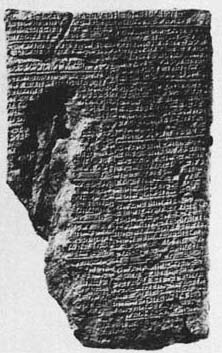Figure 6 A Babylonian tablet now in the British Museum (BM 13901)

## Box 2: A note on solving quadratic equations

If we have a quadratic equation (a problem with one unknown, involving the square of that unknown but no higher power) in its standard form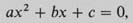then we find a solution by putting the values given for a, b and c inlo the formula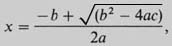which can be obtained from the standard form of the original equation through a succession of algebraic operations called completing the square.

In the particular case of a quadratic equation of the form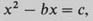into which the problem specified on the tablet fits, the procedure of completing the square goes as follows.

Take the coefficient b; halve it (½b); square that and then add it to both sides: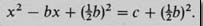Then the left hand side is the square of (x1/2b that is,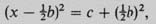so take the square root of both sides, giving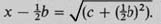Finally, add ½b to both sides to give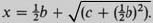## Question 5

How does the symbolic description given above compare with what the Babylonian scribe did? Approach this by considering separately: (i) what similarities there are, and (ii) what differences there are.

### Discussion

1. The sequence of instructions given by the scribe seems to follow quite closely the procedure called (in Box 2) completing the square, in terms of actions on theparticular numbers specified at the outset.

You may have noticed another similarity if you pondered the way in which the Babylonian problem was formulated. As with the stone of Question 1, the scribe seems to be labelling the unknown in an abstract, symbolic way. To see this, consider the alternative possibility that this is a realistically geometrical problem, as the words side and area seem, on the face of it, to imply. Surely the formulation of taking a side away from an area does not really make sense 10 our way of thinking about geometry (apart from conjuring up imagery of farmers ploughing up hedgerows). They are different kinds of things–taking away a hedge does not alter the numerical measure of the area that was previously enclosed. So either Babylonian geometry was quite different from ours, or they were using the terms side and area not as meaning geometrical entities particularly, but more as unknown and square of the unknown. In other words, side referred to some number to be discovered, with no connotations of where it came from or what, if anything, it measured. This is similar, perhaps, to the way we speak of x squared without necessarily imagining a square.

2. I expect you observed that there are major notational differences. It is worth noting that these are of three sorts: our ‘x’ for unknown, our ‘b’ and ‘c’ for fixed numbers, where we do not want to be specific about what they are, and all the other symbols (+, √, =, and so on). The latter seem fairly harmless translations of Babylonian words and operations in this case.

It has been argued above that our x and their side are conceptually more similar than they may first sight. But in changing their numbers into our ‘b’ and ‘c’ we effected a rather dramatic conceptual change. There is nothing in the Babylonian text paralleling our formula, a structure in which all the contributions of the original coefficients are still evident.

But bear in mind that our ‘formula’ only makes sense through our understanding the conventions about the order in which the operations it embodies are to be performed. Given a formula like √(c + (½b)2), we are taught to interpret it as, ‘square ½b, then add c, before taking the square root…’, which is beginning to sound like Babylonian instructions again. Indeed, the parallel is all the more marked in the computational techniques developed over the last couple of decades Solving a quadratic equation on a pocket calculator or computer involves carrying out a sequenced program of operations closely mirroring the instructions on the Babylonian tablet, even down to pushing the ‘square root’ button at just the stage the scribe would have leant over to consult his square root tables.

All the problem texts that have solutions are of this sort, apparently instructing about a general approach through particular instances. In some cases, all the answers on a particular tablet turn out to be the same, which seems a clear indication that it was the journey rather than the destination that mattered. This is confirmed by details within the calculations. For instance, sometimes a number is explicitly multiplied by 1, which seems pointless until one realises that, in general, it might be some number other than 1 at that stage. This serves as a reminder that some multiplication is to be done there.

MA290_2